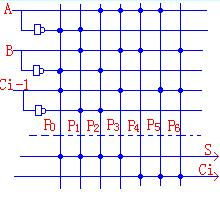# PLA and its application

When the ROM is used, since its address decoder is fixed, the function cannot be simplified, thereby occupying more area of â€‹â€‹the ROM chip. To solve this problem, we use a device that can simplify the function - PLA

PLA
The PLA is a logic device that can be programmed. It can program logic "and", "or" arrays, and use PLA to easily implement combinatorial logic and timing logic.
Example: 1 Implementing a binary full adder with PLA The simplest logical expression of the full adder is (see Chapter 4, Section 3):

S=ABCi-1+ABCi-1+ABCi-1+ABCi-1
Ci=AB+ACi-1+BCi-1
There are seven product terms in the formula, which are:
P0=ABCi-1 P1=ABCi-1 P2=ABCi-1 P3=ABCi-1
P4=AB P5=ACi-1 P6=BCi-1
According to the above, it can be concluded that the array structure diagram of the PLA full adder is as shown in Fig. (1).

It can be seen that PLA is equivalent to a ROM with both "and" or "array" programmable.